# Optimal design parameters of air suspension systems for semi-trailer truck. Part 2: results and discussion

## Nguyen Van Tuan1, Le Van Quynh2, Vi Thi Phuong Thao3, Le Quang Duy4

1, 2, 4Faculty of Automotive and Power Machinery Engineering, Thai Nguyen University of Technology, Thai Nguyen, Vietnam

3Faculty of International Training, Thai Nguyen University of Technology, Thai Nguyen, Vietnam

2Corresponding author

Vibroengineering PROCEDIA, Vol. 33, 2020, p. 147-152. https://doi.org/10.21595/vp.2020.21563
Received 25 June 2020; accepted 9 August 2020; published 19 October 2020

47th International Conference on Vibroengineering in Timisoara, Romania, October 19-20, 2020

Copyright © 2020 Nguyen Van Tuan, et al. This is an open access article distributed under the Creative Commons Attribution License, which permits unrestricted use, distribution, and reproduction in any medium, provided the original work is properly cited.
Views 272
Abstract.

Based on the results of model and algorithm in Part1 for searching the optimal design parameters of vehicle suspensions using genetic algorithm, in Part 2, the simulation results with MATLAB Simulink combined with an optimal program are written to search the optimal design parameters of vehicle air suspension systems in two optimal conditions. The optimal results indicate that the DLC values at all axles of vehicle respectively reduce by 9.09 %, 10.71 %, 11.11%, 10.81 %, 8.82 % (in Case 1) and 14.29 %, 19.23 %, 20.00 %, 17.14 %, 12.12 % (in Case 2) in comparison with the original air suspension systems when vehicle moves on the ISO class C road surface at $v=$ 20 m/s and full load. All these things indicate that using the genetic algorithm optimization method does not only reduce the tire dynamic loads acting on road surface but also improves the ride comfort. Finally, the optimum performance of vehicle suspension systems is considered and reassessed under different operating conditions. The evaluation results indicate that the optimum performance of the air suspension systems has better potential to improve the road friendliness which provides a ride comfort for road surfaces and vehicle.

Highlights
• The geometric parameters of the air springs of suspension systems are optimized by genetic algorithm.
• The design parameters of the air suspension systems are optimized by genetic algorithm.
• The simulation results with MATLAB Simulink combined with an optimal program are written to search the optimal design parameters of vehicle air suspension systems in two optimal conditions.

Keywords: semi-trailer truck, air suspension system, dynamic load coefficient, genetic algorithm, optimal parameters, road friendliness.

#### 1. Introduction

In order to improve the performance of the air suspension system for reducing the negative impact on the road surface, the control and optimization methods for air suspension systems are discussed in some of the following studies such as the control methods: the genetic LQG and PID control were used to control an air suspension system , the performance of the air suspension system of heavy trucks was analyzed with semi-active fuzzy control  and the two-bag air suspension system for heavy-duty vehicles was analyzed using the multi-body vehicle model , the optimization technique available in OptiY with SIMULINK simulation was used to search the optimal parameters of air spring of suspension system using vehicle dynamic model with 2 d.o.f , The air suspension system with independent height and stiffness tuning was analyzed and the geometric parameters of air spring were optimized , the glowworm swarm optimization proportional-integral-derivative controlling algorithm was designed to optimize magnetorheological damper for air suspensions , the vehicle suspension parameters of non-linear air spring was analyzed and optimized by using the multi-objective optimization method , and the optimization of suspension geometric parameters was analyzed and optimized using a double-loop multi-objective particle swarm optimization algorithm (DL-MOPSO) when the vehicle operates under various driving conditions . The optimal parameters of the suspension systems as well as drum’s isolation system were found out using genetic algorithm (GA) [9, 10]. Based on the results of model and algorithm in Part 1, the rest of this paper of Part 2 is organized as follows: Optimization of suspension parameters and discussion are presented in Section 2. Section 3 presents the efficiency of the optimal design parameters compared with the original design parameters of air suspension systems under the different vehicle operating conditions and the conclusions are given in Section 4.

#### 2. Optimization of suspension parameters and discussion

In order to find the optimal design parameters for the air suspension systems of the articulated truck semi-trailer, Matlab/Simulink software is used to simulate the half-vehicle dynamic model with a set of parameters of the articulated truck semi-trailer in Table 1 when the vehicle moves on the ISO class C road surface at 20 m/s and full load.

Table 1. Parameters of the 5-axle semi-trailer truck

 Parameters Values Parameters Value Parameters Values ${k}_{t1}$ / (N.m-1) 647500 ${k}_{k}$ / (N.m-1) 2000000 ${I}_{c}$ / kg.m2 2056 ${k}_{t2}$ / (N.m-1) 1185000 ${k}_{s}$ / (N.m-1) 28500 ${l}_{1}$ / m 2.741 ${k}_{t3}$ / (N.m-1) 1185000 ${c}_{k}$ / (N.s.m-1) 200000 ${l}_{2}$ / m 2.229 ${k}_{t4}$ / (N.m-1) 1185000 ${c}_{s}$ / (N.s.m-1) 9200 ${l}_{3}$ / m 3.334 ${k}_{t5}$ / (N.m-1) 1185000 ${m}_{a1}$ / kg 584 ${l}_{4}$ / m 1.330 ${c}_{t1}$ / (N.s.m-1) 258.50 ${m}_{a2}$ / kg 735 ${l}_{5}$ / m 2.960 ${c}_{t2}$ / (N.s.m-2) 325.00 ${m}_{a3}$ / kg 735 ${l}_{6}$ / m 5.620 ${c}_{t3}$ / (N.s.m-1) 325.00 ${m}_{a4}$ / kg 735 ${l}_{7}$ / m 2.685 ${c}_{t4}$ / (N.s.m-1) 325.00 ${m}_{a5}$ / kg 735 ${l}_{8}$ / m 1.115 ${c}_{t5}$ / (N.s.m-1) 325.00 ${m}_{b1}$ / kg 3783 ${l}_{9}$ / m 0.235 ${c}_{1}$ / (N.s.m-1) 11270 ${m}_{b2}$ / kg 24800 ${l}_{10}$ / m 1.200 ${k}_{c1}$ / (N.m-1) 33860 ${m}_{c}$ / kg 1208 ${\alpha }_{2,3}$ 0.0708 ${k}_{c2}$ / (N.m-1) 33860 ${m}_{s}$ / kg 116 ${\alpha }_{4,5}$ 0.0481 ${c}_{c1}$ / (N.s.m-1) 3650 ${I}_{b1}$ / kg.m2 45819 ${\beta }_{2,3}$ 0.0223 ${c}_{c2}$ / (N.s.m-1) 3650 ${I}_{b2}$ / kg.m2 195820 ${\beta }_{4,5}$ 0.0399

Operation parameters of genetic algorithm as follows: The population size is 100; The number of generations is 200; Elitism: best seven individuals from each generation were chosen for creation of next population; Mutation: random and adaptive mutations were used; Crossover: just neighboring individuals were crossed; and selection: random . A genetic algorithm program in Matlab are called by Simulink module function using the sim function, the simulation results are calculated after the calculation of the population of the objective function value. The optimal values of the parameters of air suspension systems are obtained by genetic algorithms method in comparison with the original design parameters of air suspension systems with two cases, as shown in Table 2 and Table 3.

Table 2. GA optimization results in Case 1

 Air spring suspension parameters Initial design parameters GA optimization Case 1 Optimal design of geometrical parameters of air spring suspension systems ${A}_{02}$ / m2 0.085 0.010 ${A}_{03}$ / m2 0.085 0.016 ${V}_{02}$ / m3 0.028 0.003 ${V}_{03}$ / m3 0.028 0.005 ${p}_{02}$ / MPa 0.692 0.428 ${p}_{03}$ / MPa 0.692 0.528 ${A}_{04}$ / m2 0.092 0.041 ${A}_{05}$ / m2 0.092 0.032 ${V}_{04}$ / m3 0.03 0.014 ${V}_{05}$ / m3 0.03 0.011 ${p}_{04}$ / MPa 0.492 0.254 ${p}_{05}$ / MPa 0.492 0.260

Table 3. GA optimization results in Case 2

 Case 2 Optimal design of parameters of air suspension systems ${A}_{02}$ / m2 0.085 0.013 ${A}_{03}$ / m2 0.085 0.021 ${V}_{02}$ / m3 0.028 0.004 ${V}_{03}$ / m3 0.028 0.007 ${p}_{02}$ / MPa 0.692 0.243 ${p}_{03}$ / MPa 0.692 0.304 ${A}_{04}$ / m2 0.092 0.069 ${A}_{05}$ / m2 0.092 0.032 ${V}_{04}$ / m3 0.03 0.023 ${V}_{05}$ / m3 0.03 0.011 ${p}_{04}$ / MPa 0.492 0.287 ${p}_{05}$ / MPa 0.492 0.337 ${c}_{2}$ / (N.s.m-1) 26500 53000 ${c}_{3}$ / (N.s.m-1) 26500 66300 ${c}_{4}$ / (N.s.m-1) 38000 24700 ${c}_{5}$ / (N.s.m-1) 38000 20900

The optimal results of the DLC values at 1st, 2nd, 3rd, 4th and 5th axles of vehicle in comparison with those original results with two cases are shown in Table 4. It is indicated from Table 4 that the DLC values of the optimal design parameters of air suspension systems at all axles of vehicle respectively reduce by 9.09 %, 10.71 %, 11.11 %, 10.81 %, 8.82 % (in Case 1) and 14.29 %, 19.31 %, 20.00 %, 17.14 %, 12.12 % (in Case 2) in comparison with the original air suspension systems.

All these things indicate that using this genetic algorithm optimization method does not only reduce the tire dynamic loads acting on road surface but also improves the ride comfort.

Table 4. Comparison of optimized results

 Parameters and indexes DLCt1 DLCt2 DLCt3 DLC4 DLCt5 Case 1 Optimal design of geometrical parameters of air spring suspension systems Before optimization 0.024 0.031 0.030 0.041 0.037 After optimization 0.022 0.028 0.027 0.037 0.034 Decrease % 9.09 10.71 11.11 10.81 8.82 Case 2 Optimal design of parameters of air suspension systems Before optimization 0.024 0.031 0.030 0.041 0.037 After optimization 0.021 0.026 0.025 0.035 0.033 Decrease % 14.29 19.23 20.00 17.14 12.12

The comparison of optimized results of the vertical dynamic tire loads acting on road surface in time domain response at 2nd axle in case 1 and 4th axle in case 2 are show Fig. 1 and Fig. 2. The results from Fig. 1 and Fig. 2 show that the performance optimization of the air suspension systems with two cases is better than the original air suspension systems for reducing the negative impact on road surface, especially when the optimum design parameters of the air suspension systems in case 2.

#### 3. Different operating conditions of vehicle

To evaluate the performance of the optimal design parameters of the air suspension systems on road surface friendliness, the different operating conditions such as road surface roughness, vehicle speed and vehicle load [11-15] are analyzed respectively in this paper.

Fig. 1. Comparison of the vertical dynamic tire loads acting on road surface at 2nd axle in case 1 between before and after optimization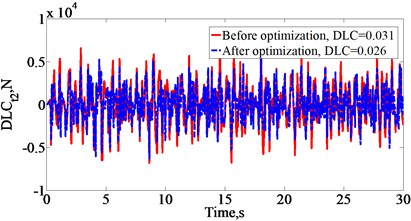Fig. 2. Comparison of the vertical dynamic tire loads acting on road surface at 4th axle in case 2 between before and after optimization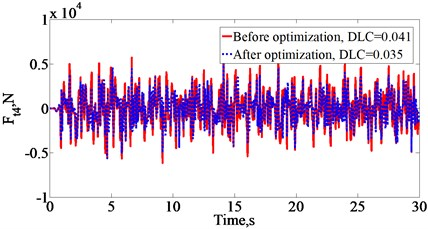#### 3.1. Effect of road surface roughness

To evaluate the performance of the optimal design parameters of the air suspension systems in comparing with the original design parameters for reducing the negative impact on road surface with two cases, five road conditions from ISO class A (very good) to ISO class E (very poor) are used when vehicle moves at the vehicle speed of 20 m/s and full load. The DLC values at 2nd and 4th axles of vehicle with three cases are shown in Fig. 3(a). Fig. 3(a) shows that the DLC values at 2nd and 4th axles of vehicle with the optimal design parameters of air suspension system in case 1 respectively reduce by 10.96 %, 10.83 %, 10.71 %, 9.92 %, 9.31 % and 10.92 %, 10.95 %, 10.81 %, 9.35 %, 9.31 % in comparison with the original air suspension systems of vehicle. Similarly, the DLC values at 2ndand 4th axles of vehicle significantly reduce by 19.19 %, 20.00 %, 19.23 %, 19.91 %, 20.38 % and 17.26 %, 17.34 %, 17.14 %, 19.67%, 20.00 %, respectively in Case 2.

#### 3.2. Effect of vehicle speed

The vehicle speeds of 5 m/s, 10 m/s, 15 m/s, 20 m/s and 25 m/s are considered to evaluate the performance of the optimal design parameters of the air suspension systems with two cases for a 5- axle semi-trailer truck when vehicle moves on the road surface condition of the ISO class C and full loaded. The DLC values at 2nd and 4th axles of vehicle with three cases are shown in Fig. 3(b), indicating that the optimal results have a significant improvement in road-friendly level in comparison with the original air suspension systems of vehicle at all vehicle speeds. Moreover, the DLC values at 2ndand 4th axles of vehicle with the optimal design parameters of air suspension system in the optimal results in case 2 are reduced by 6.14 %, 9.23 %, 7.95 % and 4.12 %, 2.31 %, 6.33 %, respectively in comparison with Case 1 when the vehicle speed increases from 10 to 15 m/s, and then to 20 m/s. The DLC values increase or decrease in abnormal manner in each of the vehicle speed because the vehicle speed affects the product of vehicle speed and the cutoff values of the roughness spatial frequency .

Fig. 3. Comparing of the DLC values at 2nd and 4th axles of vehicle with three cases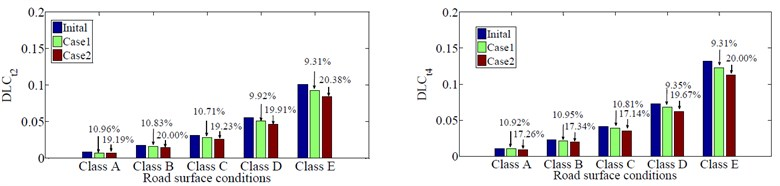a) Effect of road surface roughness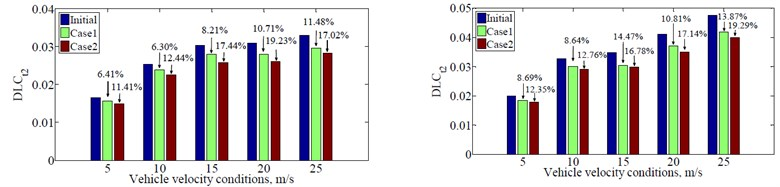b) Effect of vehicle speed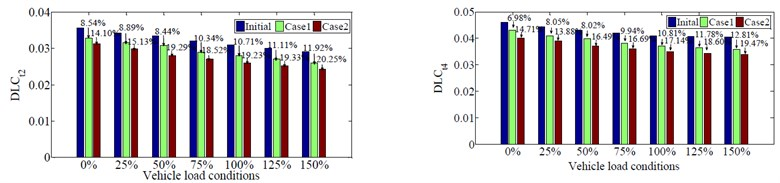#### 3.3. Effect of vehicle load

To continue evaluating the optimal design parameters of the air suspension systems in comparing with the original design parameters for reducing the negative impact on road surface with two cases, seven load conditions of vehicle from 0 % (empty load) to 150 % (over 150 %) are used when vehicle moves on the road surface condition of the ISO class C and the vehicle speed of 20 m/s. The DLC values at 2nd and 4th axles of vehicle with three cases are shown in Fig. 3(c). Fig. 3(c) shows that the DLC values at 2nd and 4th axles of vehicle with the optimal design parameters of air suspension system in case 2 significantly reduce by 14.10 %, 15.13 %, 19.29 %, 18.52 %, 18.31 %, 19.33 %, 20.25 % and 14.71 %, 13.88 %, 16.49 %, 16.69 %, 17.14 %, 18.86 %, 19.41 %, respectively in comparison with the original air suspension systems of vehicle. Moreover, the values of DLC at 2nd and 4th axles of vehicle increase the fastest when vehicle move under 50 % load condition and this problem has a negatively effect on road surface. However, when the vehicle's load increases over 75 % vehicle load, the values of DLC at 2nd and 4th axles of vehicle reduce gradually, which has a benefit effect on road surface. However, it has no benefit on the durability of the part of vehicle and the safe movement of vehicle .

#### 4. Conclusions

In part 2 of this study, the simulation results by using MATLAB Simulink combined with an optimal program is written to solve the optimization problem of nonlinear air suspension parameters in two optimal conditions. The obtained the optimization results show that the DLC values of the optimal design parameters of air suspension systems at all axles of vehicle reduce in comparison with the initial air suspension systems of vehicle when vehicle moves on the ISO class C road surface at 20 m/s and full load. Especially, the DLC values of the optimal design parameters of air suspension systems at 1st, 2nd, 3rd 4th and 5th axles of vehicle considering case 2 respectively reduce by 4.76 %, 7.69 %, 8.00 %, 5.71 % and 3.03 % in comparison in Case 1. Finally, the optimum performance of vehicle suspension systems is considered and evaluated again under different operating conditions and the obtained optimization results indicated that the optimum performance is the significant reduction the tire loads and road surface damage. The study results can be used for the searchers, vehicle manufacturers and traffic managers for further considerations during design as well as perfect design in the design of vehicle air suspension systems.

#### Acknowledgements

This research was supported financially by Thai Nguyen University of Technology, TNUT, Viet Nam.

1. Tang Chuan Yin, Li Yun Gong Research on air suspension system based on genetic LQG and PID control. International Conference on Optics, Electronics and Communications Technology, 2017. [Publisher]
2. Nguyen V., Zhang J., et al. Performance analysis of air suspension of heavy truck with semi-active fuzzy control. Journal of Southeast University (English Edition), Vol. 33, Issue 2, 2017, p. 159-165. [Search CrossRef]
3. Tajima J., Momiyama F., Yuhara N. A new solution for two-bag air suspension system with leaf spring for heavy-duty vehicle. Vehicle System Dynamics, Vol. 44, Issue 2, 2006, p. 107-138. [Publisher]
4. Abid H. J., Chen J., Nassar A. A. Equivalent air spring suspension model for quarter-passive model of passenger vehicles. International Scholarly Research Notices, Vol. 2015, 2015, p. 974020. [Publisher]
5. Karimi Eskandary P., Khajepour A., Wong A., Ansari M. Analysis and optimization of air suspension system with independent height and stiffness tuning. International Journal of Automotive Technology, Vol. 17, Issue 5, 2016, p. 807-816. [Publisher]
6. Xiao P., Gao H., Shi P., Niu L. Research on air suspension with novel dampers based on glowworm swarm optimization proportional-integral-derivative algorithm. Advances in Mechanical Engineering, Vol. 10, Issue 8, 2018, https://doi.org/10.1177/1687814018791710. [Publisher]
7. Li J. H., He J., Li X. H. Multi-Objective optimization of vehicle air suspension based on Simulink-Mfile mixed programming. Applied Mechanics and Materials, Vol. 509, 2014, p. 63-69. [Publisher]
8. Yikai Chen, Sen Huang, Lloyd Davis, et al. Optimization of geometric parameters of longitudinal-connected air suspension based on a double-loop multi-objective particle swarm optimization algorithm. Applied Sciences, Vol. 8, Issue 9, 2018, p. 1454. [Publisher]
9. Goga V., Kľúčik M. Optimization of vehicle suspension parameters with use of evolutionary computation. Procedia Engineering, Vol. 48, 2012, p. 174-179. [Publisher]
10. Quynh L. V., Thao V. T. P., Phong T. T. Optimal design parameters of drum’s isolation system for a double-drum vibratory roller. Vibroengineering Procedia, Vol. 31, 2020, p. 74-79. [Publisher]
11. Le V. Q. Comparing the performance of suspension system of semi-trailer truck with two air suspension systems. Vibroengineering Procedia, Vol. 14, 2017, p. 220-226. [Publisher]
12. Long L. X., Hong T. T., et al. Performance analysis of the hydro-pneumatic suspension system of heavy truck. International Journal of Mechanical Engineering and Technology, Vol. 9, Issue 13, 2018, p. 1128-1139. [Search CrossRef]
13. Van Cuong B., Van Quynh L., Long L. X. Influence of heavy truck operating condition on dynamic load coefficient. Advances in Engineering Research and Application, 2018, p. 372-379. [Publisher]
14. Long L. X., Quynh L. V., Cuong B. V. Study on the influence of bus suspension parameters on ride comfort. Vibroengineering Procedia, Vol. 21, 2018, p. 77-82. [Publisher]
15. Van Quynh L. Influence of semi-trailer truck operating conditions on road surface friendliness. Vibroengineering Procedia, Vol. 16, 2017, p. 67-72. [Publisher]
16. Shi X. M., Cai C. S. Simulation of dynamic effects of vehicles on pavement using a 3D interaction model. Journal of Transportation Engineering, Vol. 135, Issue 10, 2009, p. 736-744. [Publisher]
17. Le V. Q., Zhang J., Liu X., Wang Y. Nonlinear dynamic analysis of interaction between vehicle and road surfaces for 5-axle heavy truck. Journal of Southeast University, Vol. 27, Issue 4, 2011, p. 405-409. [Search CrossRef]

#### Cited By

 Lecture Notes in Networks and Systems Le Xuan Long, Dang Viet Ha, Nguyen Van Tuan, Vu Thanh Niem, Vu Thi Hien 2023 Lecture Notes in Networks and Systems Ta Tuan Hung, Le Xuan Long, Nguyen Van Tuan, Hoang Anh Tan, Vu The Truyen 2023 Heliyon Truong Manh Hung 2022